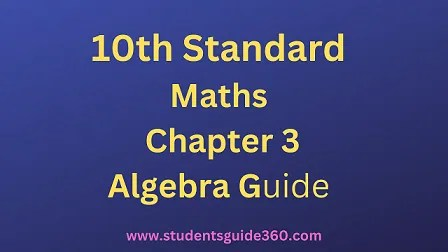# 10th Maths Guide Chapter 3. Algebra Exercise 3.9

10th Standard Maths Chapter 3 Algebra Samacheer Kalvi Guide Exercise 3.9 Book Back Answers Solutions. TN 10th SSLC Samacheer Kalvi Guide. 10th All Subject Guide – Click Here. Class 1 to 12 All Subject Book Back Answers – Click Here## 10th Maths Guide Chapter 3. Algebra Exercise 3.9

### 1. Determine the quadratic equations, whose sum and product of roots are

• (i) -9, 20
• (ii) 5/3, 4
• (iii) −3/2, -1
• (iv) -(2 – a)2, (a + 5)2

Solution:
If the roots are given, general form of the quadratic equation is x2 – (sum of the roots) x + product of the roots = 0.
(i) Sum of the roots = -9
Product of the roots = 20
The equation = x2 – (-9x) + 20 = 0
⇒ x2 + 9x + 20 = 0

(ii) Sum of the roots = 5/3
Product of the roots = 4
Required equation = x2 – (sum of the roots)x + product of the roots
= 0
⇒ x2 – 53x + 4 = 0
⇒ 3×2 – 5x + 12 = 0

(iii) Sum of the roots = (−3/2)
(α + β) = −32
Product of the roots (αβ) = (-1)
Required equation = x2 – (α + β)x + αβ = 0
x2 – (−32)x – 1 = 0
2×2 + 3x – 2 = 0

(iv) α + β = – (2 – a)2
αβ = (a + 5)2
Required equation = x2 – (α + β)x – αβ = 0
⇒ x2 – (-(2 – a)2)x + (a + 5)2 = 0
⇒ x2 + (2 – a)2x + (a + 5)2 = 0

### 2. Find the sum and product of the roots for each of the following quadratic equations

• (i) x2 + 3x – 28 = 0
• (ii) x2 + 3x = 0
• (iii) 3 + 1/a=10/a2
• (iv) 3y2 – y – 4 = 0

(i) x2 + 3x – 28 = 0
Sum of the roots (α + β) = -3
Product of the roots (α β) = -28

(ii) x2 + 3x = 0